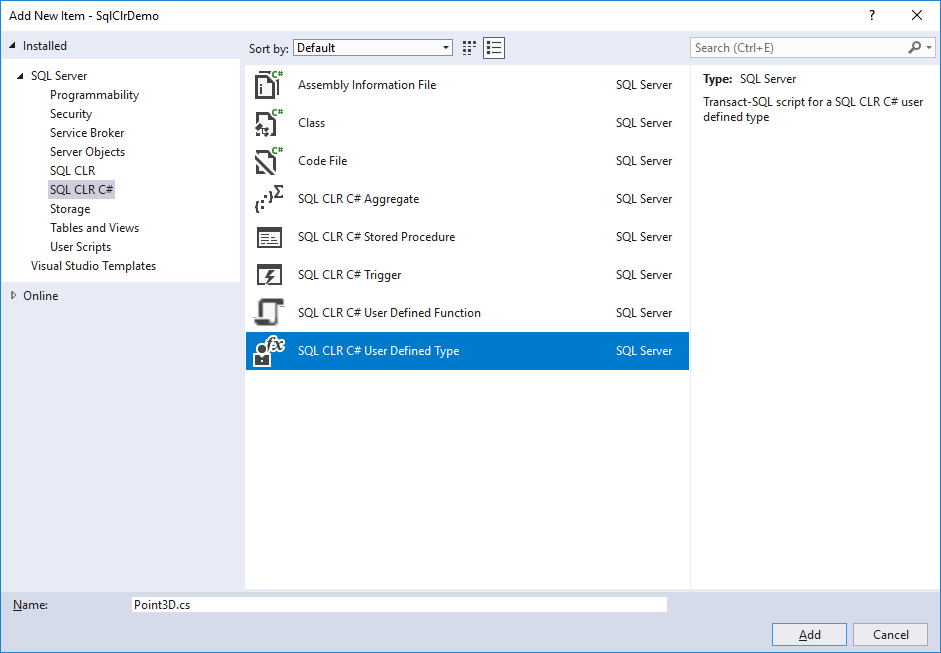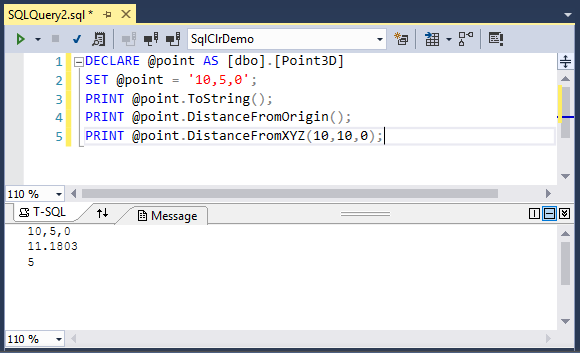# SQL CLR CLR User-Defined Types

30% OFF - 9th Anniversary discount on Entity Framework Extensions until December 15 with code: ZZZANNIVERSARY9

The CLR User-Defined Types (UDTs) extend the scalar type system and enabling storage of CLR objects in a SQL Server database, and provide the ability to define complex structured types.

To create a CLR user-defined type, select Project > Add New Item menu option.Select the SQL CLR C# from the list on the left pane, and choose SQL CLR C# User-Defined Type in the middle pane. It will add the following code for you.

``````using System;
using System.Data;
using System.Data.SqlClient;
using System.Data.SqlTypes;
using Microsoft.SqlServer.Server;

[Serializable]
[Microsoft.SqlServer.Server.SqlUserDefinedType(Format.Native)]
public struct Point3D: INullable
{
public override string ToString()
{
// Replace with your own code
return string.Empty;
}

public bool IsNull
{
get
{
return _null;
}
}

public static Point3D Null
{
get
{
Point3D h = new Point3D();
h._null = true;
return h;
}
}

public static Point3D Parse(SqlString s)
{
if (s.IsNull)
return Null;
Point3D u = new Point3D();
return u;
}

// This is a place-holder method
public string Method1()
{
return string.Empty;
}

// This is a place-holder static method
public static SqlString Method2()
{
return new SqlString("");
}

// This is a place-holder member field
public int _var1;

//  Private member
private bool _null;
}
``````

Let's change this code by adding X, Y and Z properties and also add some basic functions to calculate the distance from origin or distance from any specific point.

``````using System;
using System.Data;
using System.Data.SqlClient;
using System.Data.SqlTypes;
using System.Text;
using Microsoft.SqlServer.Server;

[Serializable]
[Microsoft.SqlServer.Server.SqlUserDefinedType(Format.Native)]
public struct Point3D: INullable
{
// Properties
public bool IsNull { get; private set; }
public double X { get; set; }
public double Y { get; set; }
public double Z { get; set; }

public override string ToString()
{
if (this.IsNull)
return "NULL";
else
{
StringBuilder builder = new StringBuilder();
builder.Append(X);
builder.Append(",");
builder.Append(Y);
builder.Append(",");
builder.Append(Z);
return builder.ToString();
}
}

public static Point3D Null
{
get
{
Point3D p = new Point3D();
p.IsNull = true;
return p;
}
}

[SqlMethod(OnNullCall = false)]
public static Point3D Parse(SqlString s)
{
if (s.IsNull)
return Null;
Point3D p = new Point3D();
string[] xyz = s.Value.Split(",".ToCharArray());
p.X = double.Parse(xyz);
p.Y = double.Parse(xyz);
p.Z = double.Parse(xyz);

return p;
}

// Distance from origin to Point method.
[SqlMethod(OnNullCall = false)]
public Double DistanceFromOrigin()
{
return DistanceFromXYZ(0.0, 0.0, 0.0);
}

// Distance from point to the specified point method.
[SqlMethod(OnNullCall = false)]
public Double DistanceFromPoint(Point3D pFrom)
{
return DistanceFromXYZ(pFrom.X, pFrom.Y, pFrom.Z);
}

// Distance from point to the specified x, y and z values method.
[SqlMethod(OnNullCall = false)]
public Double DistanceFromXYZ(double iX, double iY, double iZ)
{
return Math.Sqrt(Math.Pow(iX - X, 2.0) + Math.Pow(iY - Y, 2.0) + Math.Pow(iZ - Z, 2.0));
}
}
``````

To create the CLR user-defined type in the database, you can use Visual Studio Publish option or execute the following T-SQL.

``````CREATE TYPE [dbo].[Point3D]
EXTERNAL NAME [SqlClrDemo].[Point3D]
``````

Let's test the user-defined type by running the following T-SQL

``````DECLARE @point AS [dbo].[Point3D]
SET @point = '10,5,0';
PRINT @point.ToString();
PRINT @point.DistanceFromOrigin();
PRINT @point.DistanceFromXYZ(10,10,0);
``````

It will produre the following output.